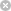RTXI  2.1DirectFormIir Member List

This is the complete list of members for DirectFormIir, including all inherited members.

 Coeff_Quan_Factor DirectFormIir private DirectFormIir(int num_numer_coeff, int num_denom_coeff, double *numer_coeff, double *denom_coeff, long coeff_quan_factor, long input_quan_factor) DirectFormIir FilterImplementation() FilterImplementation inline GetNumDenomCoeff(void) DirectFormIir GetNumNumerCoeff(void) DirectFormIir GetNumTaps(void) DirectFormIir virtual Input_Buffer DirectFormIir private Input_Quan_Factor DirectFormIir private Input_Write_Indx DirectFormIir private Num_Denom_Coeff DirectFormIir private Num_Numer_Coeff DirectFormIir private Output_Buffer DirectFormIir private Output_Quan_Factor DirectFormIir private Output_Write_Indx DirectFormIir private ProcessSample(double input_val) DirectFormIir virtual ProcessSample(long input_val) DirectFormIir virtual Quan_Denom_Coeff DirectFormIir private Quan_Numer_Coeff DirectFormIir private